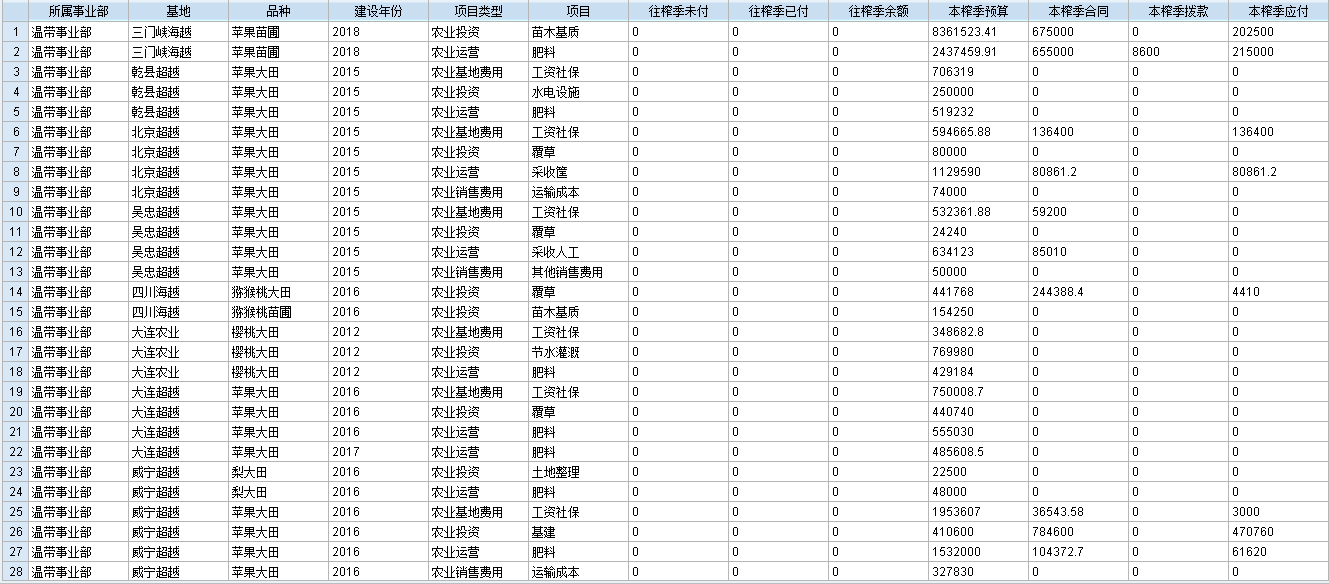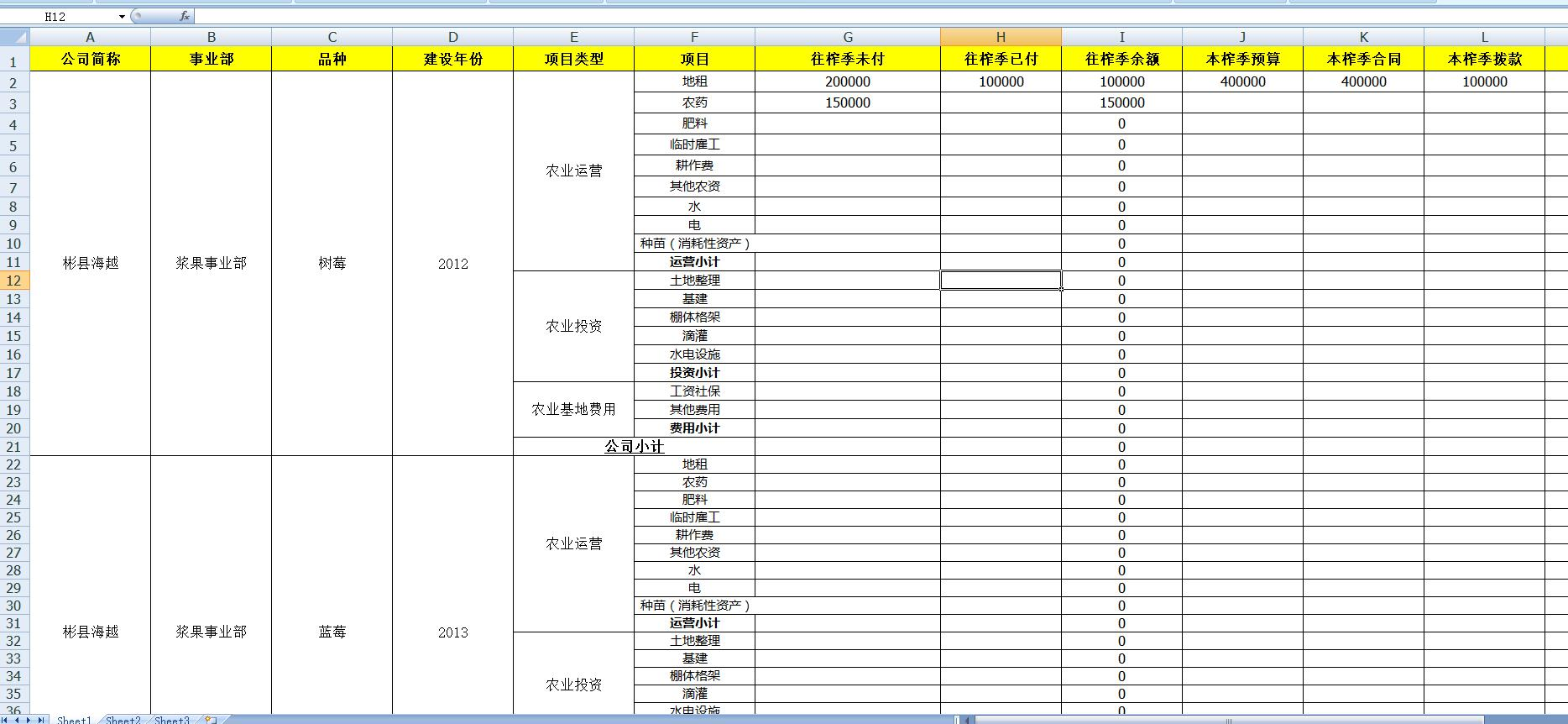oracle 添加小计，合计 5C4个回答

`````` (select name, x, y, z, (x + y+ z) as 小计 from 表)
union
(select "合计"as name, sum(x) as x, sum(y) as y, sum(z) as z, (sum(x) + sum(y)+ sum(z)) as 小计 from 表)
``````